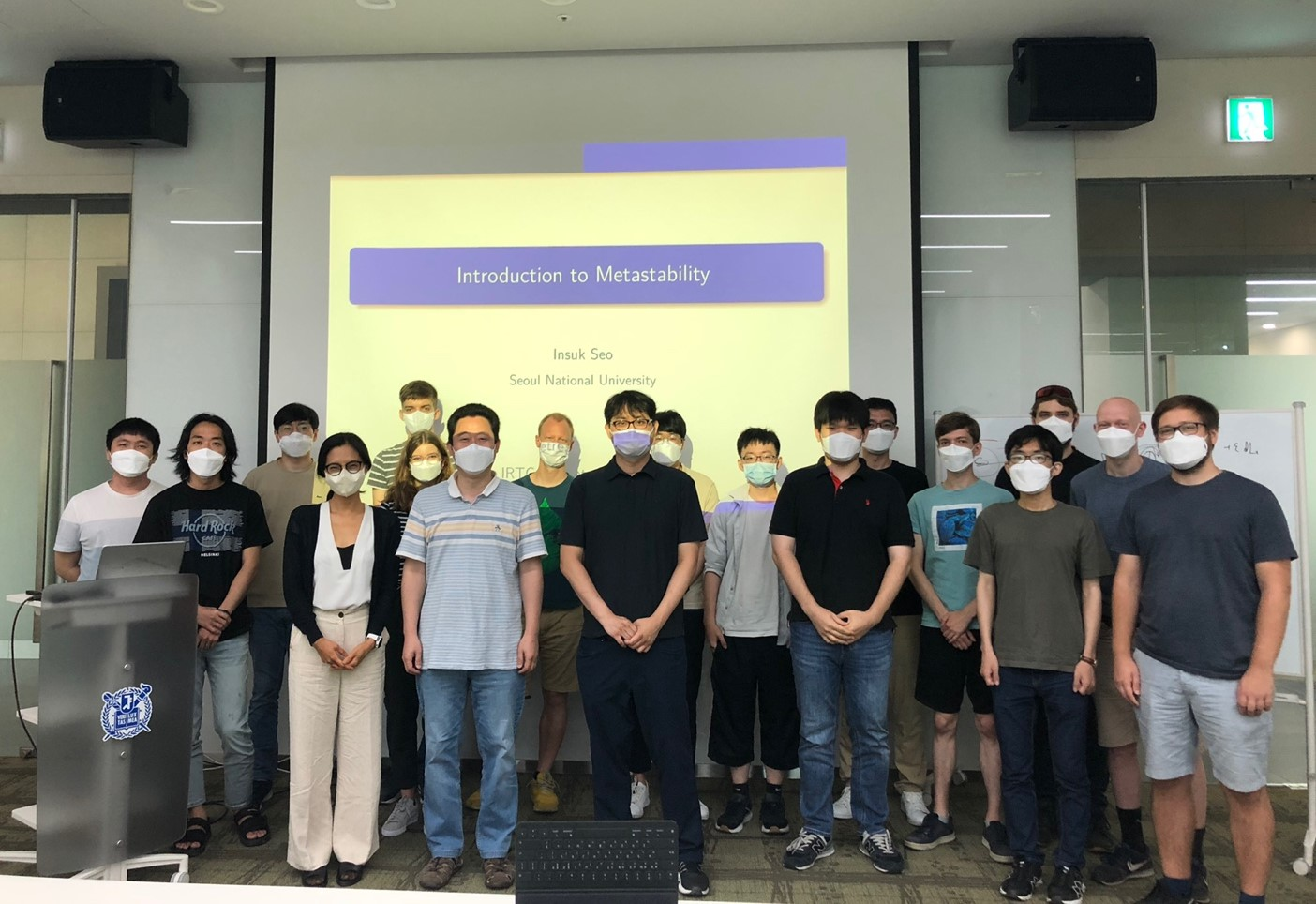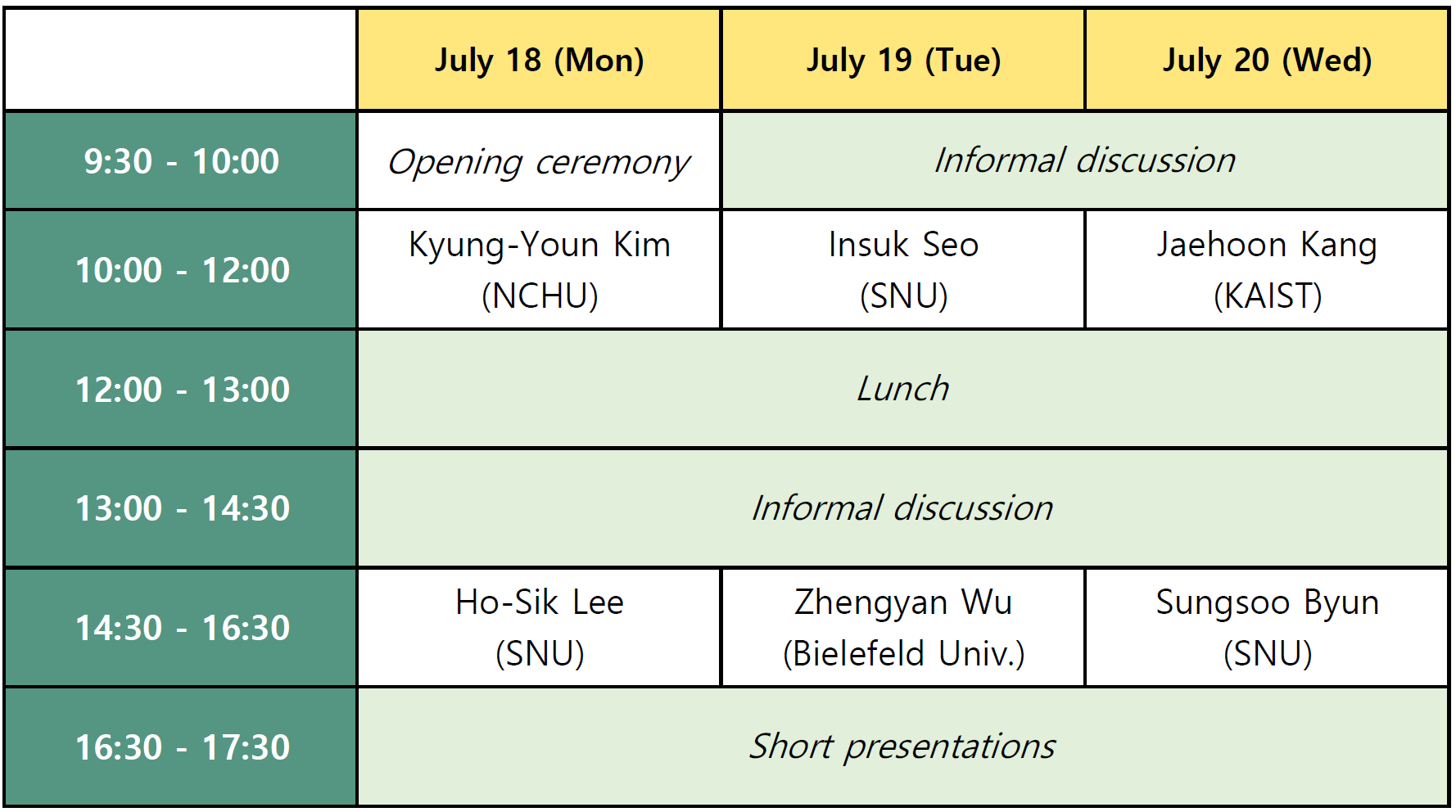IRTG Summer retreat 2022

The second Korean IRTG Summer Retreat in Mathematics

July 18 - July 20, 2022

SNU Siheung Convention Center, Gyeonggi-do## Organization

Panki Kim (Seoul National University)

## Schedule## Speakers and talks

Speaker

Title

Sungsoo Byun (KIAS)

Random Matrix Theory through the lens of the Universality Phenomenon

Jaehoon Kang (KAIST)

Subordinator and related topics

Kyung-Youn Kim (NCHU)

Introduction to Levy process

Ho-Sik Lee (SNU)

Various concepts of Solutions for elliptic PDE: Existence and Regularity

Insuk Seo (SNU)

Metastability and Resolvent equation

Zhengyan Wu (Bielefeld Univ.)

Dean-Kawasaki equation with Singular Non-local Interaction:

Well-posedness and Large deviations

## Abstracts

Sungsoo Byun (KIAS)

Abstract: Since Ginibre introduced three classes of non-Hermitian Gaussian random matrices with real, complex, and quaternion elements in 1965, non-Hermitian Random Matrix Theory (RMT) has successfully provided many applications in modelling a wide range of physical phenomena, which include quantum chromodynamics, dissipative quantum maps, and scattering in chaotic quantum systems. Furthermore, non-Hermitian RMT has inspired the development of several fields of mathematics such as free probability theory, analytic function theories of planar (skew-) orthogonal polynomials, and the theory of Painlevé transcendents.

In this talk, I will introduce various topics in non-Hermitian RMT focusing on the universality phenomenon with the goal to provide an overall picture of the RMT community. The topics include the integrable structures of non-Hermitian random matrices, the universality of the global and local eigenvalue statistics, and the real eigenvalue problems of real asymmetric random matrices.

Jaehoon Kang (KAIST)

Abstract: We will discuss subordinator and related topics in probabilistic potential theory. In particular, I will introduce recent results on estimates of transition density functions for subordinate Brownian motions and time fractional equations.

Kyung-Youn Kim (NCHU)

Abstract: In this lecture, we introduce the definition and basic properties of Levy processes. We explain infinite divisibility and Levy-Khintchine formula.

Ho-Sik Lee (SNU)

Abstract: In this talk, I consider and introduce many different kinds of solutions such as weak solution, very weak solution, viscosity solution, Solutions Obtained as Limits of Approximations (SOLA), entropy solution, renormalized solutions for elliptic equations. I briefly explain the history and background of appearing such solutions, and then I deal with the existence and regularity issues of such solutions under the suitable setting of PDE.

Insuk Seo (SNU)

Abstract: Lecture 1: Metastability of Stochastic Systems

In this lecture, we introduce a phenomenon known as metastability and discuss several famous stochastic models that exhibiting metastability phenomenon (e.g., small random perturbation of dynamical systems, interacting particle systems with sticky interaction, and Ising model). Then, we explain several mathematical formulations of metastability.
Lecture 2: Metastability and Resolvent Equations
In the second lecture, we explain the relation between the metastability and the solution of specific form of resolvent equation associated with the generator of the underlying Markovian Metastable dynamics.

Zhengyan Wu (Bielefeld Univ.)

Abstract: This talk consists of two parts. In the first part, I will give a brief introduction to stochastic partial differential equation (SPDE), including some typical examples of SPDE. Also, some preliminaries on SPDE will be involved. In the second part, I will introduce my recent work about singular interacting Dean-Kawasaki equation. The work has two folds: well-posedness theory for conservative SPDE with singular non-local interaction in the framework of kinetic solutions, and small noise large deviations in singular scaling limits.

## Participants

 Name Affiliation Behn, Linus Bielefeld University Byun, Sungsoo Korea Institute for Advanced Study Cho, Soobin Seoul National University Grube, Florian Bielefeld University Hensiek, Thorben Bielefeld University Herrmann, Simon Bielefeld University Jöckel, Niklas Bielefeld University Jung, Jinwook Seoul National University Kang, Jaehoon KAIST Kang, Myungjoo Seoul National University Kim, Kyung-youn National Chung Hsing University Kim, Panki Seoul National University Kwak, Beomjong KAIST Lee, Ho-sik Seoul National University Lee, Jaehun Korea Institute for Advanced Study Neufeld, Leonie Bielefeld University Schefer, Waldemar Bielefeld University Seo, Insuk Seoul National University Wu, Zhengyan Bielefeld University